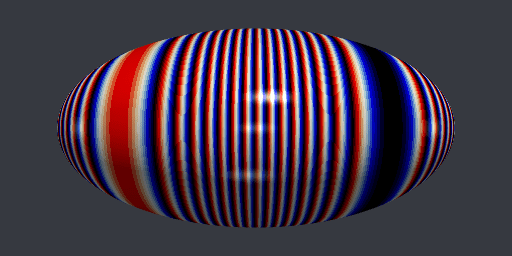# PyVista surface with dynamic colormap

Posted on January 27, 2022 by Stéphane Laurent

I’ve just done this animation with PyVista:This is a Hopf torus with the so-called flag colormap as texture, but this texture moves. Here is a link to the full code.

The trick is to normalize the scalars to $$[0, 2\pi]$$ and then to apply the map $$\sin(\cdot - t)$$ to the scalars, with $$t$$ varying from $$0$$ to $$2\pi$$. Let me show you another example, with an ellipsoid.

from math import pi, sin
import numpy as np
import pyvista as pv

mesh = pv.Sphere(theta_resolution=200, phi_resolution=200)
mesh.scale((1, 0.5, 1), inplace=True) # ellipsoid
dists = mesh.points[:,2] # we take the third coordinates for the scalars
# then we map them to [0, 2*pi]:
dists = 2*pi * (dists - dists.min()) / (dists.max() - dists.min())

t_ = np.linspace(0, 2*pi, 100, endpoint=False) # the varying parameter
for i, t in enumerate(t_):
pngfile = "pic%03d.png" % i
pltr = pv.Plotter(window_size = [512, 256], off_screen = True)
pltr.background_color = "#363940"
pltr.set_focus([0, 0, 0])
pltr.set_position((1.3, 0, 0))
mesh["dist"] = np.sin(dists - t) # set the scalars - here is the "trick"
pltr.show(screenshot = pngfile)
This code produces the png files pic000.png, …, pic099.png. Then it remains to make a gif file from them, using e.g. ImageMagick or gifski. And here is the result: